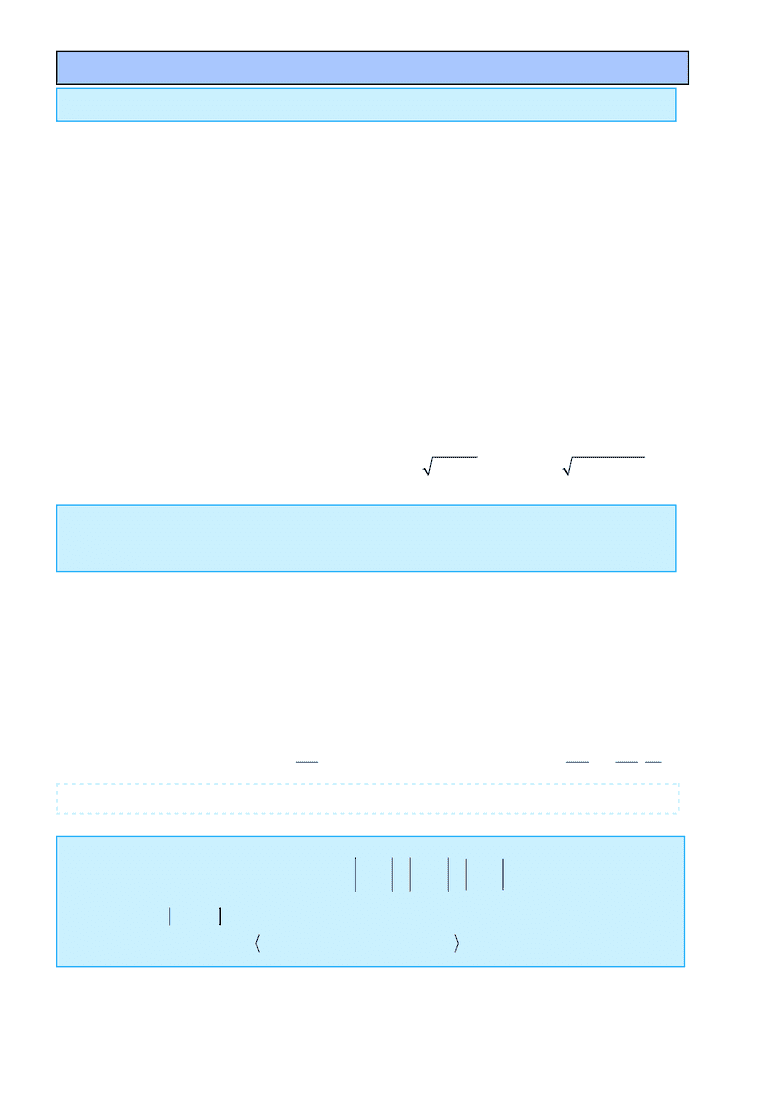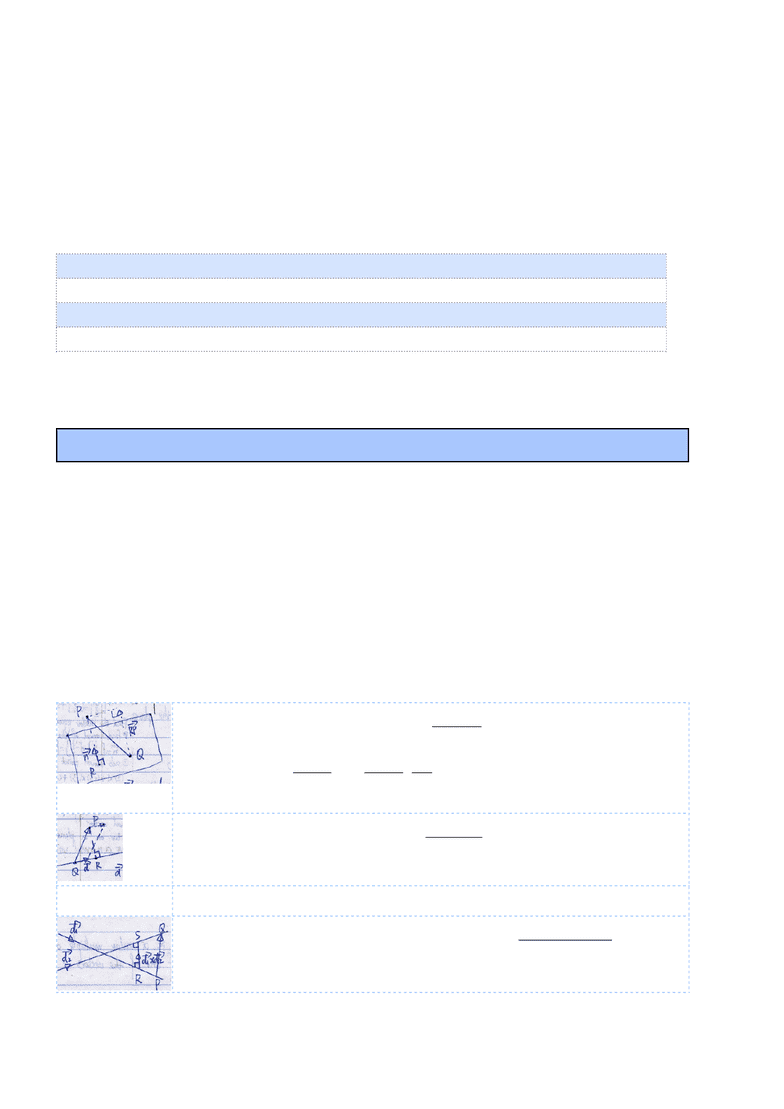# ESC103H1 Final: MAT-Linear Algebra .pdf

181 views10 pages
School
CourseLinear Algebra ESC103+MAT185
Scalar: sign+magnitude+unit Vector: direction(tip-tail)+magnitude+unit (2D/3D)
>Property of Addition: commutative v+w=w+v associative (v+w)+z=v+(w+z)
Zero: u+0=u
Negative: Given v, there is some u that satisﬁes v+u=0. we will denote this particular u by (-v)
2.Subtraction:
3.Scalar Multiplication/Product:
If 2 vectors are multiples of each other, they are parallel. u=tv
Magnitude of a vector: Euclidean Norm 2D 3D
Triangle Inequality:
|ku|=|k||u| |u|=0 <==>u=0
I.Property of Dot Product: v·v=a2+b2=|v|2 distributive u·(v+w)=u·v+u·w commutative u·v=v·u
(ku)·v=k(u·v) not associative 0·a=0
II. Geometric Interpretation: (cosine law) θ:smaller angle between v&w (less than 180°)
v·w>0 iff θ[0,π/2) v·w<0 iff θ(π/2,π] v·w=0 iff θ=π/2 (v w perpendicular)
v·wvw v·w can be + or - v·w=v·|projection of w on v|
Orthogonality: For two non-zero vectors v & w,iff v·w=0, v and w is orthogonal.
Zero vector is perpendicular to every vector.
III.Projection:
scalar projection of w onto v vector projection of w onto v
IV.Inner Product:(abstract scalar ﬁeld)
u×v orthogonal to both u & v.
Two vectors pointing same direction, there are inﬁnite number of vectors orthogonal to them.
I.Property of Cross Product: anti-commutative u×v=-v×u k(u×v)=(ku)×v=u×(kv) distributive
u×(v+w)=u×v+u×w (u+v)×w=u×w+v×w
u×0=0×u=0 not associative u×(v×w)(u×v)×w
Vector
Vector Algebra
!
v+!
w=a
b
+c
d
=a+c
b+d
!
0=0
0
!
v=a
b
!
v!
w=!
v+(!
w)=ac
bd
k!
v=ka
kb
|!
v|=a2+b2+c2
|!
v+!
w| | !
v|+|!
w|
4.Dot/Scalar Product: 2D 3D
dot product is the scalar
!
v!
w=ac +bd
!
v!
w=ac +bd +cf
!
v!
w=vw cos
θ
!
a
!
b=a1b1+a2b2+... +anbn
!
v!
w=|!
v|| !
w| cos
θ
comp!
v(!
w)=
!
w!
v
|!
v|
proj!
v(!
w)=
!
w!
v
|!
v|2!
v=
!
w!
v
|!
v|
!
v
|!
v|
Proof:
5.Cross/Vector Product:
an: right-hand rule
cross product is the vector (only deﬁned for vectors in R3)
!
v×!
w=
ˆ
iˆ
jˆ
k
a b c
d e f
=ˆ
ib c
e f ˆ
ja c
d f +ˆ
ka b
d e
=
bf ec
af +dc
ae bd
!
v×!
w=!
anvw sin
θ
!
a×
!
b=a2b3a3b2,a3b1a1b3,a1b2a2b1
Carrie Yan
Unlock document

This preview shows pages 1-3 of the document.
Unlock all 10 pages and 3 million more documents.Linear Algebra ESC103+MAT185
Right Hand Rule: direction of u×v
4 ﬁngers pointing at the ﬁrst vector(u) then curl to the second(v), thumb points to their cross product.
index-v, middle-u, thumb-u×v
II.Geometric Interpretation:(Lagrange’s identity) angle between 2 vectors θ
|u×v|2=|u|2|v|2-(u·v)2=|u|2|v|2-|u|2|v|2cos2θ=|u|2|v|2sin2θ so, |u×v|=|u||v|sinθ
Parallel: For two non-zero vectors v & w,iff v×w=0, v and w is parallel.
III.Area of parallelogram determined by u and v is length of cross product u×v
Area of Parallelogram=height×base=|u||v|sinθ (two sides u & v, height=|v|sinθ)
Volume of parallelepiped determined by u,v,w is absolute value of scalar triple product
Volume of Parallelepiped=height×area of base=|u·(v×w)|
4.Division by a vector is not deﬁned.
Vector Equation of a line:
P0(x0,y0,z0) known point on the line d: direction vector
Vector Equation of a plane:
d1&d2 not parallel
Scalar Equation of a plane:
n: normal vector and
How we see/draw:
central projection
parallel projection
|!
v×!
w|=|!
v|| !
w| sin
θ
Scalar Triple Product u·(v×w)=w·(u×v)=v(w×u)=-u·(w×v)=-w·(v×u)=-v(u×w)
Vector Triple Product(Lagrange Formula/back-cab rule) u×(v×w)=v(u·w)-w(u·v)
Lines & Planes
x
y
z
=
x0
y0
z0
+t
!
d
!
P=
!
P
0+t
!
d
x
y
z
=
x0
y0
z0
+t
!
d1+s
!
d2
!
P=
!
P
0+t
!
d1+s
!
d2
a(xx0)+b(yy0)+c(zz0)=0
ax +by +cz =ax0+by0+cz0
!
n=
!
d1×
!
d2=
a
b
c
P
0P
! "!!
=
xx0
yy0
zz0
P
0P
! "!!
!
n=0
P arbitrary point in space, Q
arbitrary point on plane, R
projection of P on plane
Distance from Point to Plane:
|RP
! "!!
|=|!
nQP
! "!!
|
|"
n|
RP
! "!!
=proj!
n(QP
" !""
)=
!
nQP
! "!!
|"
n|2!
n=
!
nQP
! "!!
|"
n|
!
n
|!
n|
Distance between 2 lines:
|RS
! "!
|=proj!
d1×
!
d2
(PQ
" !""
)=| (
!
d1×
!
d2)PQ
! "!!
|
|
"
d1×
!
d2|
Distance between 2 points:
P
1P
2
! "!!!
=OP
2
! "!!
OP
1
! "!!
Distance from Point to Line:
|RP
! "!!
|=|QP
! "!!
×
!
d|
|
!
d|
Carrie Yan
Unlock document

This preview shows pages 1-3 of the document.
Unlock all 10 pages and 3 million more documents.Linear Algebra ESC103+MAT185
orthogonal projection
m×n Matrix m rows n columns
2.Scalar Multiplication:
3.Multiplication: nAm×mBp = nCp inner dimensions must match
Properties: A(B+C)=AB+AC (AB)C=A(BC) ABBA
Linear Transformation: a function that maps a vector to a vector with properties, L(kv)=kL(v) and L(u
+v)=L(u)+L(v)
identity transformation: I(u)=u zero transformation: 0(u)=0
Composition of two transformation: linear transformation C as A followed by B,C(u)=B(A(u))
Mc=Mb·Ma
d
Inverse and Determinant
M-1M=1 determinant: ad-bc when =0, no inverse
Reduced Normal Form(RNF)
A matrix is in RNF if: the ﬁrst non-zero entry in each row is a 1; the other entries in the columns
containing these leading 1’s are zero; the leading 1’s move to the right as we move down the rows;
any zero rows are collected at the bottom.
Elementary Operations--Gaussian Elimination
Intercharge two equations (two rows of augmented matrix)
Multiply one equation(row) by a non-zero constant
Add a multiple of one equation to another equation(row)
Elementary Matrices: we can associate matrices with three elementary operations.
A linear system:
Consistent System: inverse
i) Unique Solution(every variable in RNF is a leading variable)
ii) Inﬁnite Solution(at least one free variable)
Solutions to AX=b with m equations and n variables(total number of variables), if rank is r(number of
leading variables), the number of free variables is n-r (number of free variables).
Homogeneous System: AX=0 trivial solution X=0
Inconsistent System: No solution least square problem
error vector (when AXB)
Normal Equations:
least squares solution
Matrix
M1=1
db
c a
!
E=A!
xb=
e1
e2
.
.
em
!
E=e1
2+...em
2
AT(bA!
x)=
!
0
ATbATA!
x=0
ATb=ATA!
x
!
x=(ATA)1ATb
Carrie Yan
Unlock document

This preview shows pages 1-3 of the document.
Unlock all 10 pages and 3 million more documents.